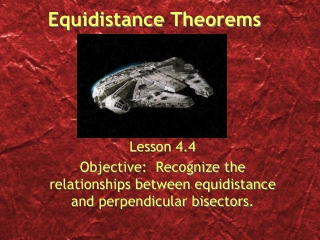Download PresentationEquidistance Theorems

# Equidistance Theorems

Download Presentation## Equidistance Theorems

- - - - - - - - - - - - - - - - - - - - - - - - - - - E N D - - - - - - - - - - - - - - - - - - - - - - - - - - -
##### Presentation Transcript

1. Equidistance Theorems Lesson 4.4 Objective: Recognize the relationships between equidistance and perpendicular bisectors.

2. Hi graham

3. The term distance has special meaning. • Definition:The distance between two objects is the length of the shortest path joining them. • Postulate:A line segment is the shortest path between two points. S R The distance between points R and S is the length of RS, or RS.

4. Equidistant: If two points P and Q are the same distance from a third point X, then X is said to be equidistant from P and Q. X P Q

5. Definition: Perpendicular bisector of a segment is the line that bisects and is perpendicular to the segment. Theorem 24: If two points are each equidistant from the endpoints of a segment, then the two points determine the perpendicular bisector of that segment.

6. Theorem 25: Any point on the perpendicular bisector is equidistant from the end points. If AD and GE are both perpendicular and bisect each other, then any point on either line is equidistant from the opposite endpoints. G A D M E

7. Given • Given • 2 points each equidistant from the endpoints of a seg. determine the  bisector of the seg. • A point on the  bisector of a seg is equidistant from the endpoints of the seg. • 1. AB  AD • 2. BC  CD • AC  bis. BD • BE  ED